# Long Division - Definition with Examples

The Complete K-5 Math Learning Program Built for Your Child

• 40 Million Kids

Loved by kids and parent worldwide

• 50,000 Schools

Trusted by teachers across schools

• Comprehensive Curriculum

Aligned to Common Core

## Long Division Games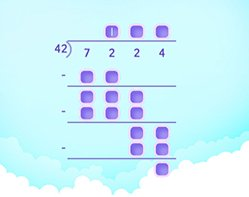Divide by 2 Digit Numbers

Divide 4 digit numbers by 2 digit numbers where the division does not leave any remainder. You will start off by estimating quotients.

Covers Common Core Curriculum 5.NBT.6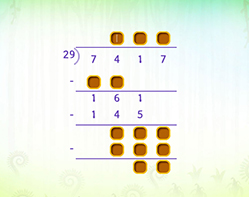Divide by 2 Digit Numbers with Remainder

Divide 4 digit numbers by 2 digit numbers. Start off with estimating quotients which comes in handy while dividing by 2 digit numbers.

Covers Common Core Curriculum 5.NBT.6

## What is long division?

In math, long division is a method used for dividing large numbers into groups or parts.
Long division helps in breaking the division problem into a sequence of easier steps. Just like all division problems, a large number, which is the dividend, is divided by another number, which is called the divisor, to give a result called the quotient and sometimes a remainder.

How do you do long division?
The long division method involves basic math operations.

To divide two numbers using this method, a tableau is drawn. The divisor is written outside the right parenthesis, while the dividend is placed within. The quotient is written above the over bar on top of the dividend.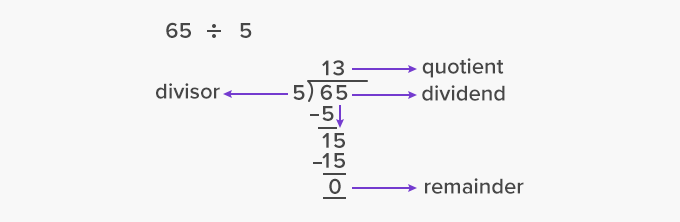Long division involves 5 steps:

 D Divide M Multiply S Subtract B Bring down R Repeat or Remainder

Here’s an example of long division with each step shown clearly.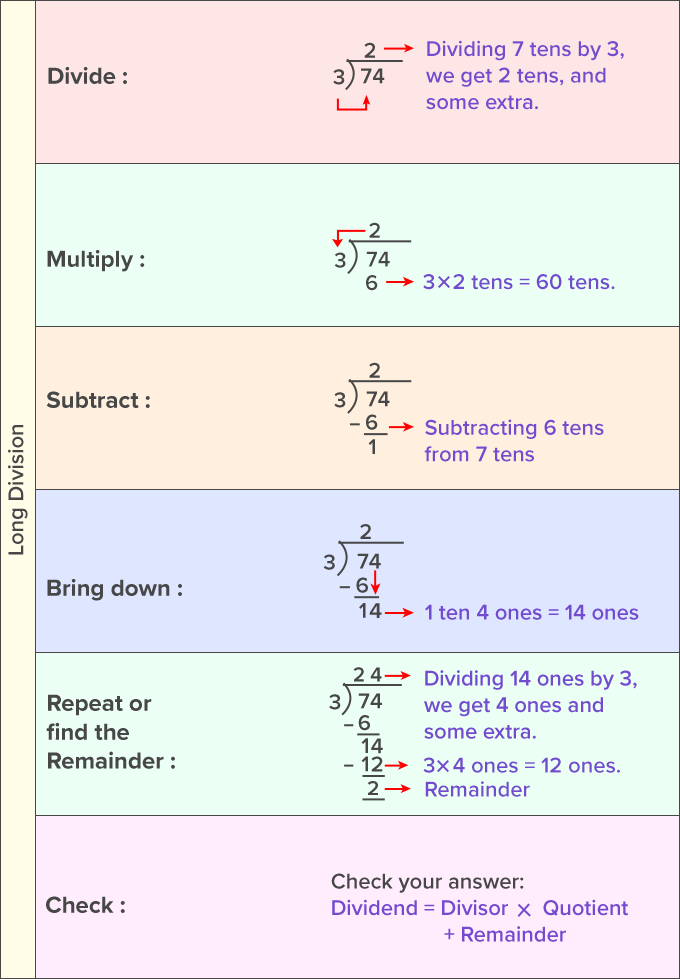The process begins with dividing or finding how many times the leftmost digit of the dividend can be divided by the divisor.
Then, the result or answer from step 1, which becomes the first digit of the quotient, is multiplied by the divisor and written under the first digit of the dividend.
Subtraction is carried out on the first digit of the dividend and the remainder is written.
The next digit of the dividend is brought down and then, the process is repeated until all the digits of the dividend are brought down and a remainder is found.

How to divide decimals using the long division method?
Long division can also be used to divide decimal numbers into equal groups. It follows the same steps as that of long division, namely, – divide, multiply, subtract, bring down and repeat or find the remainder.

Here’s an example of long division with decimals.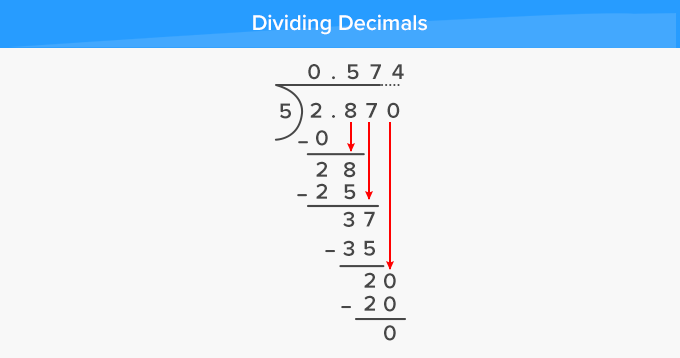Fun Facts 123454321 when divided by 11111 gives a quotient of 11111 and remainder 0.

##Let's sing!

When you have large numbers to divide,
draw a tableau for long division on the side.
Write the steps that will be your guide,
D, M, S, B and R – Abide by to long divide!

##Let's do it!

Instead of showing videos for teaching long division or handing out practice worksheets to your 4th graders, cite real-life instances wherein they can use long division method to divide.
Say, while preparing cupcakes and cookies for a bake sale in school, you can ask your child to calculate the number of batches in which cookies or cupcakes can be prepared (basis the number of molds in a tray) if the total number of cookies and cupcakes required is given. You can also ask them to calculate the total number of cartons required if each cookie carton packs 15 cookies and the cupcake carton packs 6 cupcakes. Ask them to calculate using the long division method.

##Related math vocabulary

Won Numerous Awards & Honors